GRE Subject Test: Math : Functions of More Than Two Variables

Example Questions

Example Question #131 : Calculus

Solve for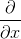: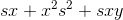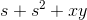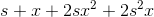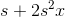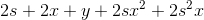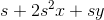Explanation:

To solve for the partial derivative, let all other variables be constants besides the variable that is derived with respect to.

In, the terms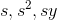are constants.

Derive as accordingly by the differentiation rules.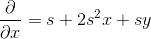Example Question #1 : Functions Of More Than Two Variables

Suppose the function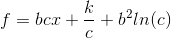.  Solve for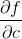.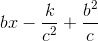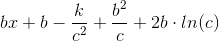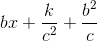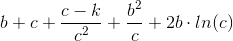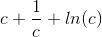Explanation:

Identify all the constants in function.

Since we are solving for the partial differentiation of variable, all the other variables are constants.  Solve each term by differentiation rules.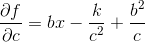Example Question #1 : Functions Of More Than Two Variables

Suppose the function.  Solve for.Explanation:

Identify all the constants in function.

Since we are solving for the partial differentiation of variable, all the other variables are constants.  Solve each term by differentiation rules.All GRE Subject Test: Math Resources